## Improving an Estimate For the Value of an Integral Using the Trapezium Rule

The trapezium rule gives an estimate for the value of an integral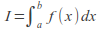as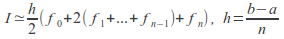with accuracy proportional to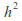so that if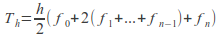and the true value of the integral is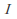then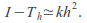We can obtain an improved estimate for the value of the integral using the trapezium rule twice with different values of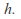Suppose that we use the trapezium rule twice with different values of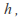obtaining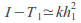(1) and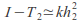(2)

(1)-(2) gives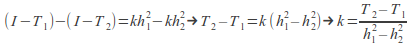Substituting back into (2) (assuming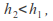so that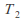is a better estimate for I) gives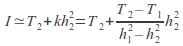For a very simple example, consider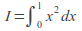Take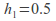and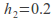so that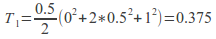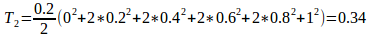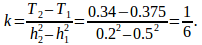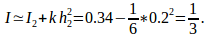In fact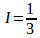exactly, so in this case the estimate is exactly right.